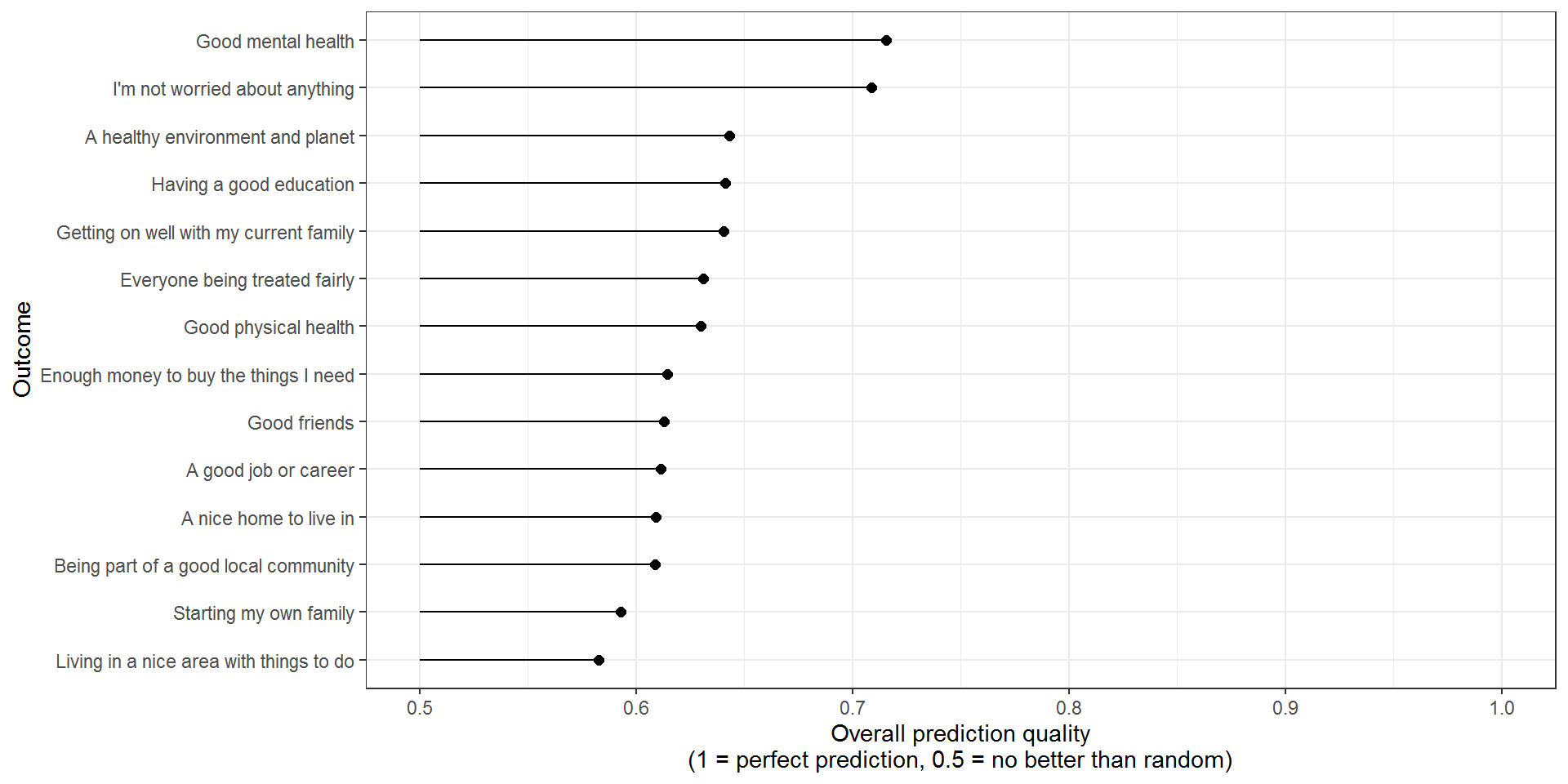# What do children worry about for their future?: Regression analysis

#### What types of characteristics are most predictive?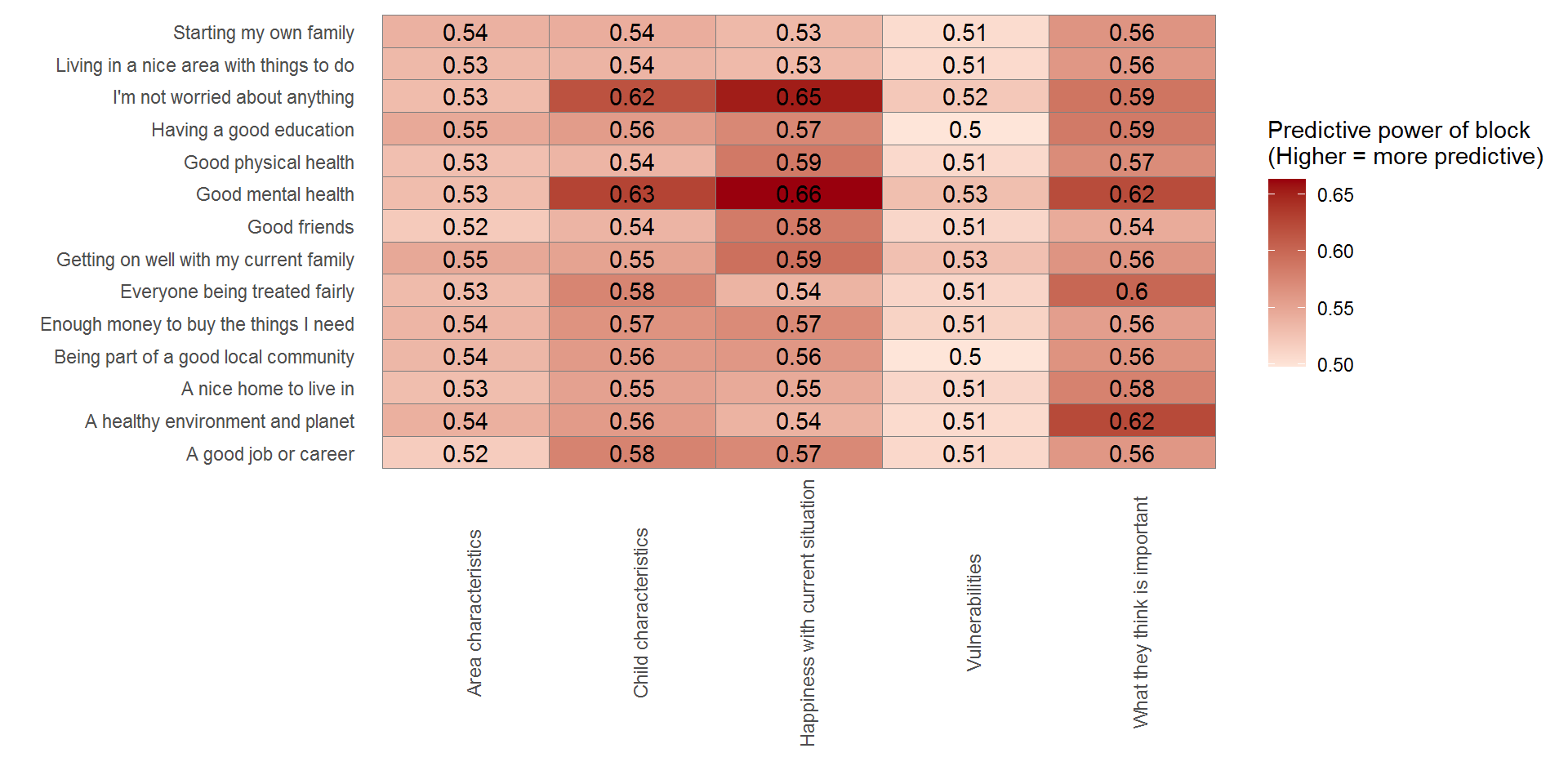#### What specific characteristics are most predictive?

Overall predictive power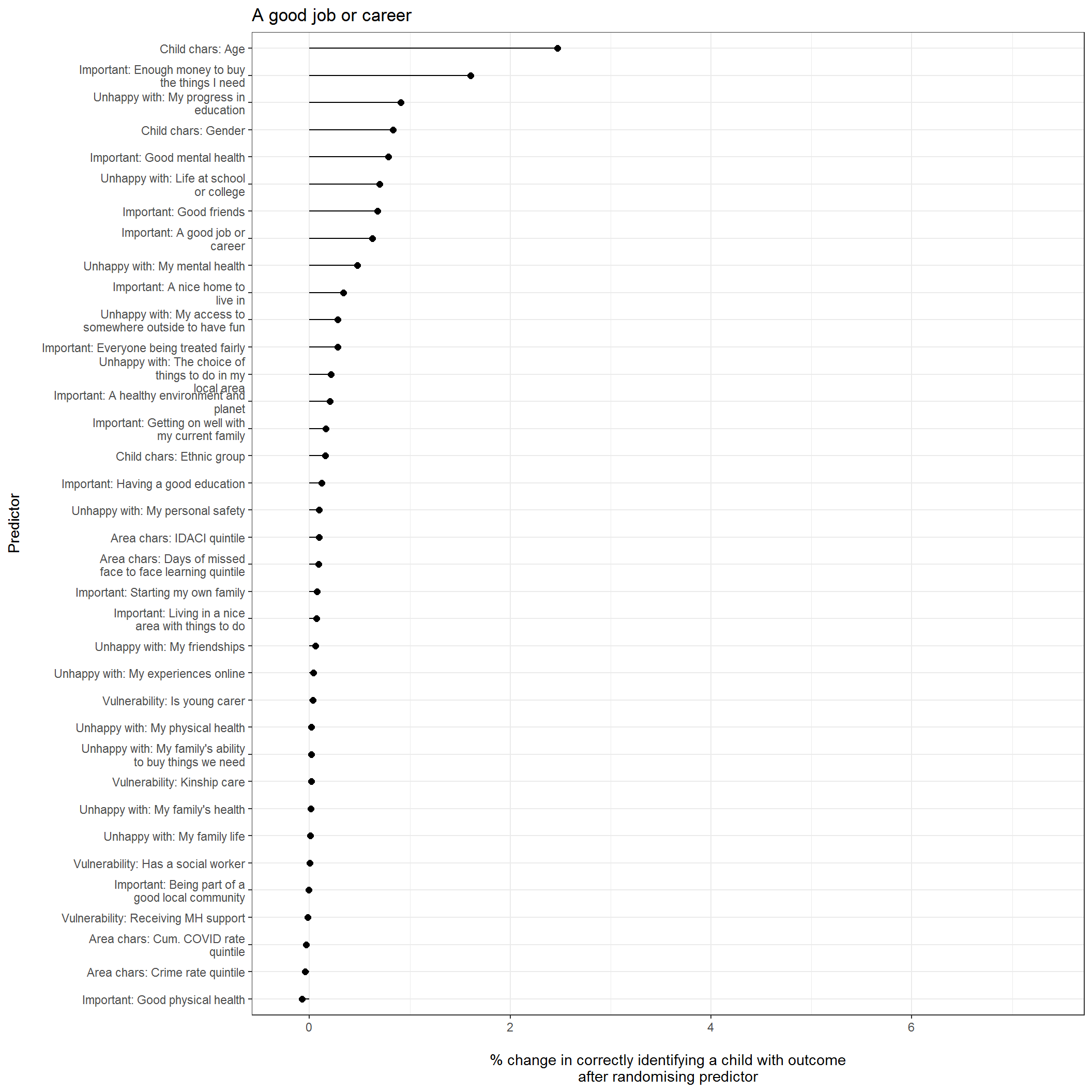Odds ratios

Table below below presents odds ratios for each predictor in the model after controlling for all other predictors included

Overall predictive power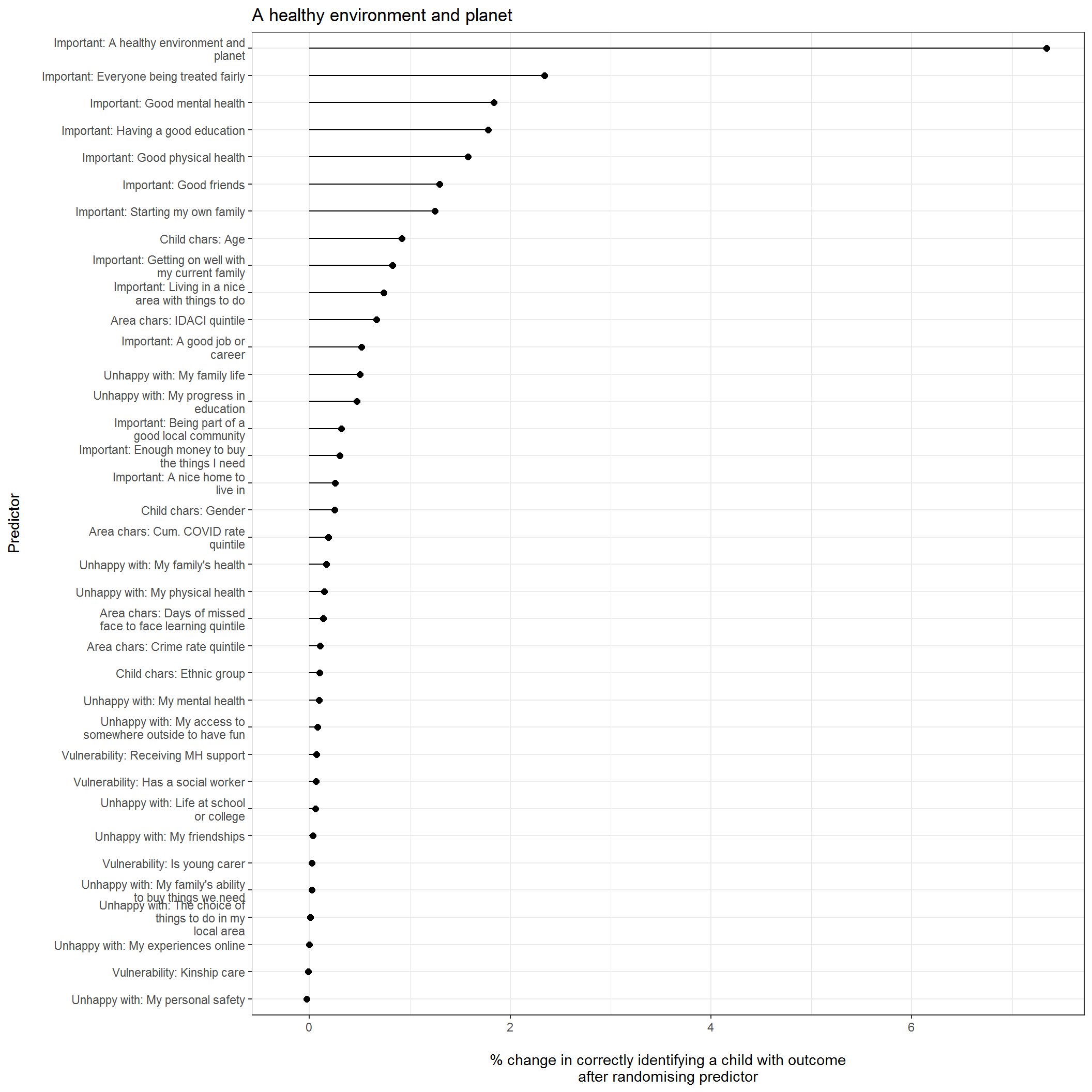Odds ratios

Table below below presents odds ratios for each predictor in the model after controlling for all other predictors included

Overall predictive power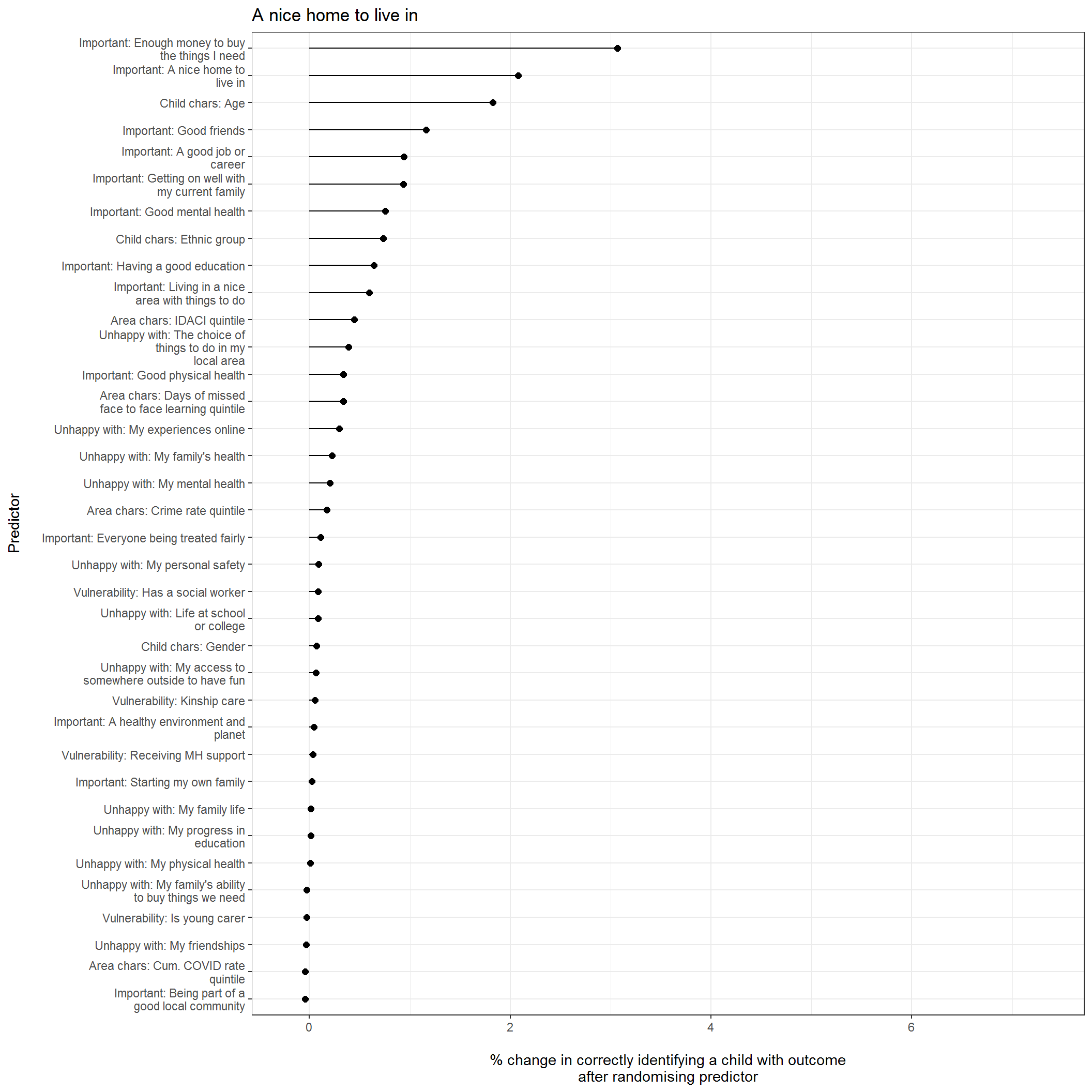Odds ratios

Table below below presents odds ratios for each predictor in the model after controlling for all other predictors included

Overall predictive power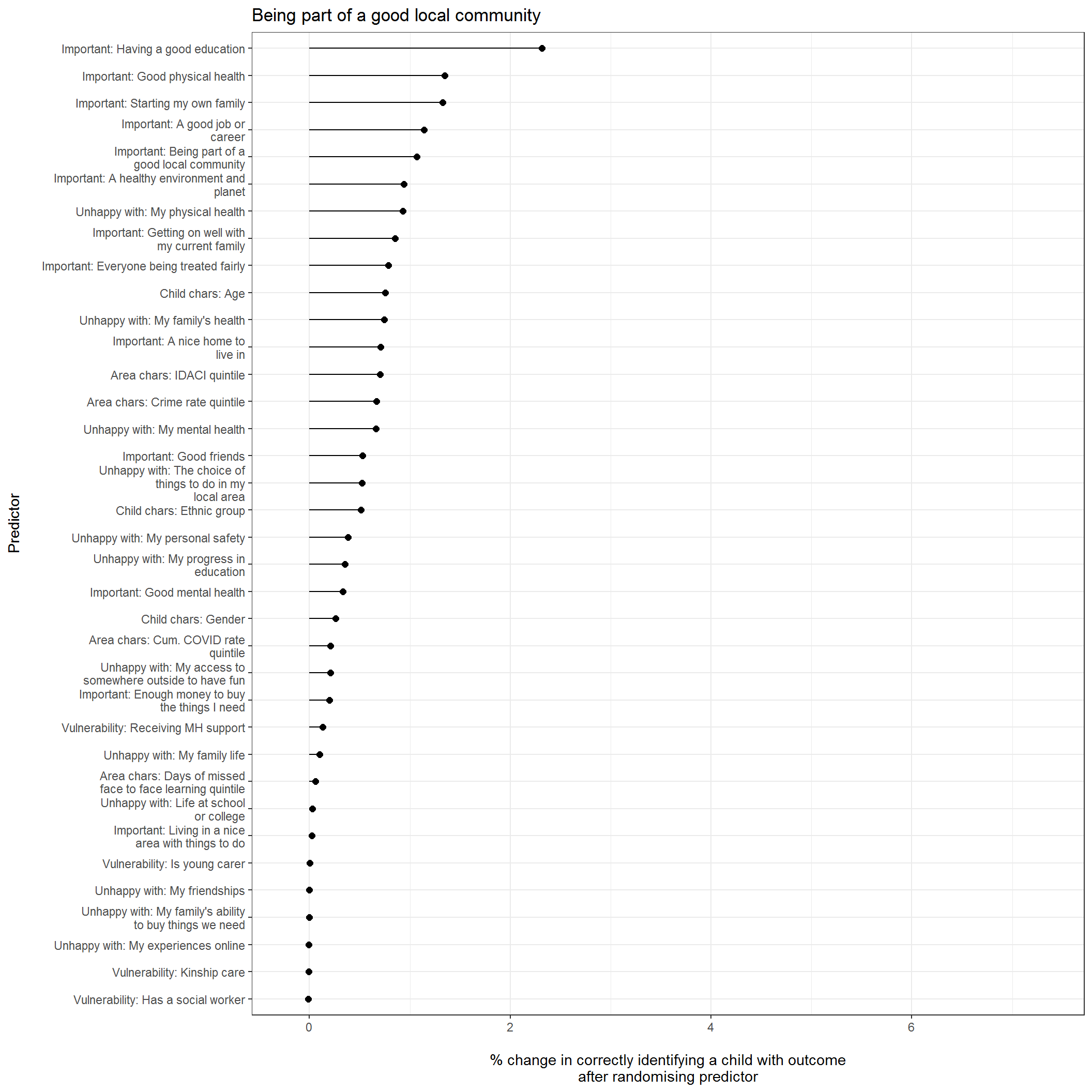Odds ratios

Table below below presents odds ratios for each predictor in the model after controlling for all other predictors included

Overall predictive power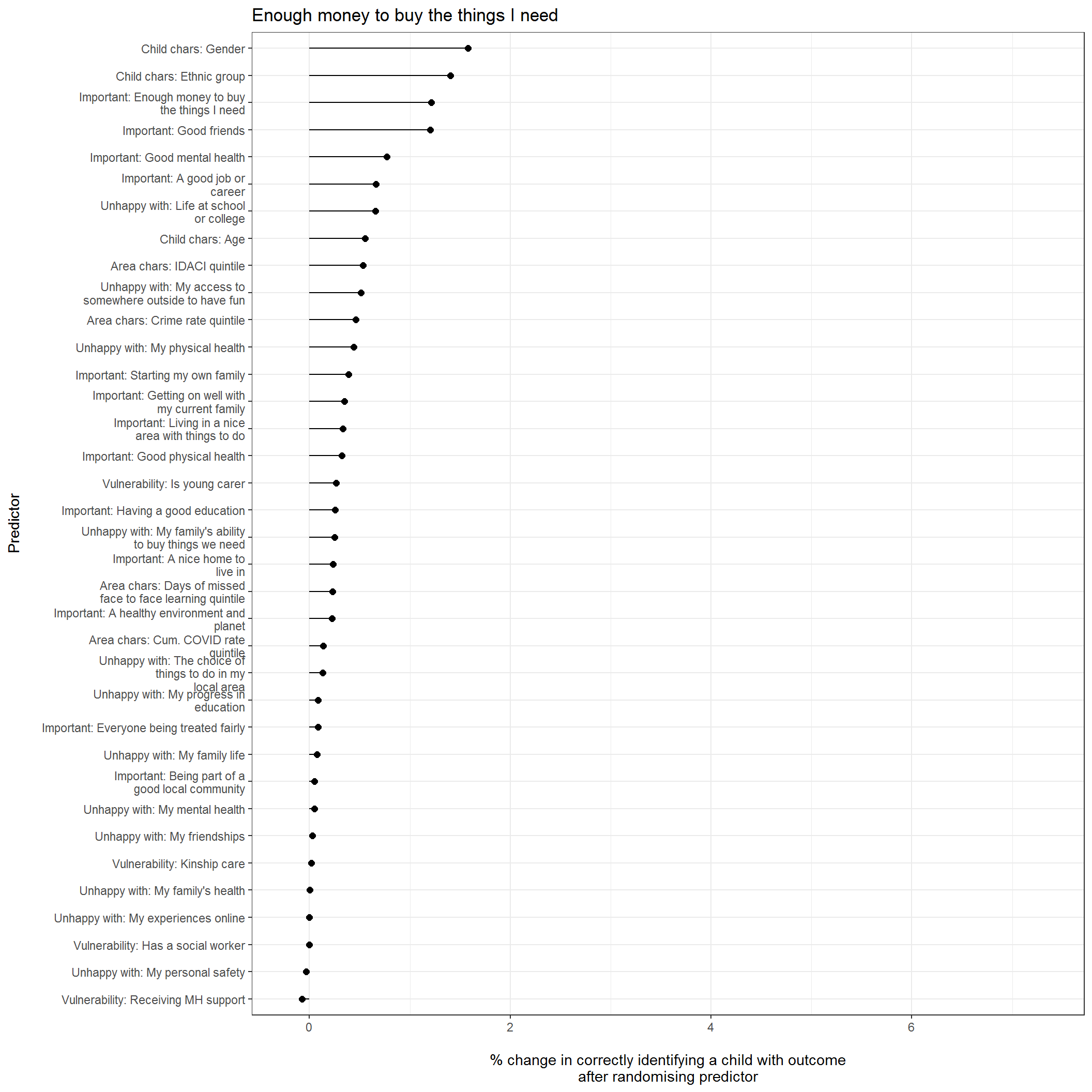Odds ratios

Table below below presents odds ratios for each predictor in the model after controlling for all other predictors included

Overall predictive power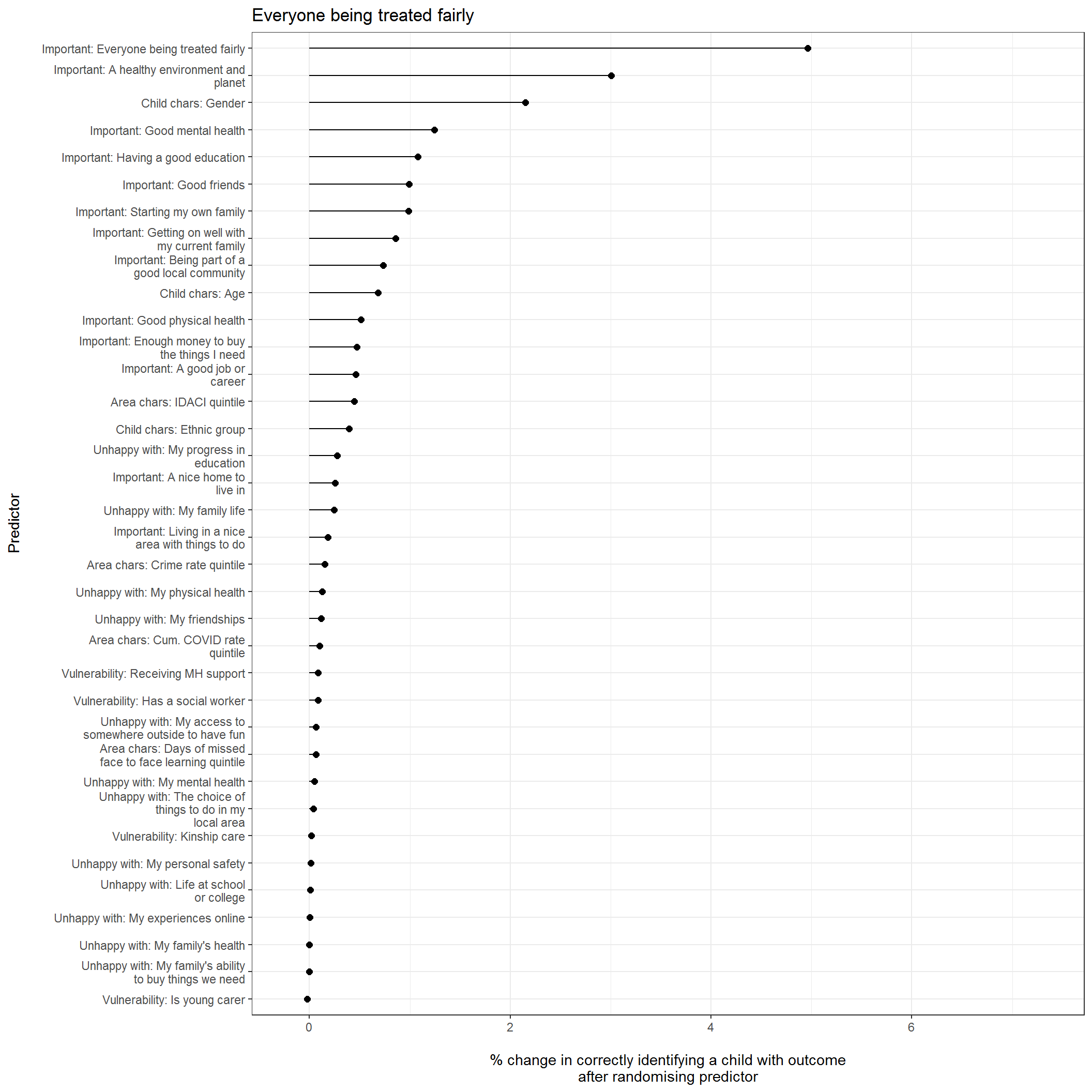Odds ratios

Table below below presents odds ratios for each predictor in the model after controlling for all other predictors included

Overall predictive power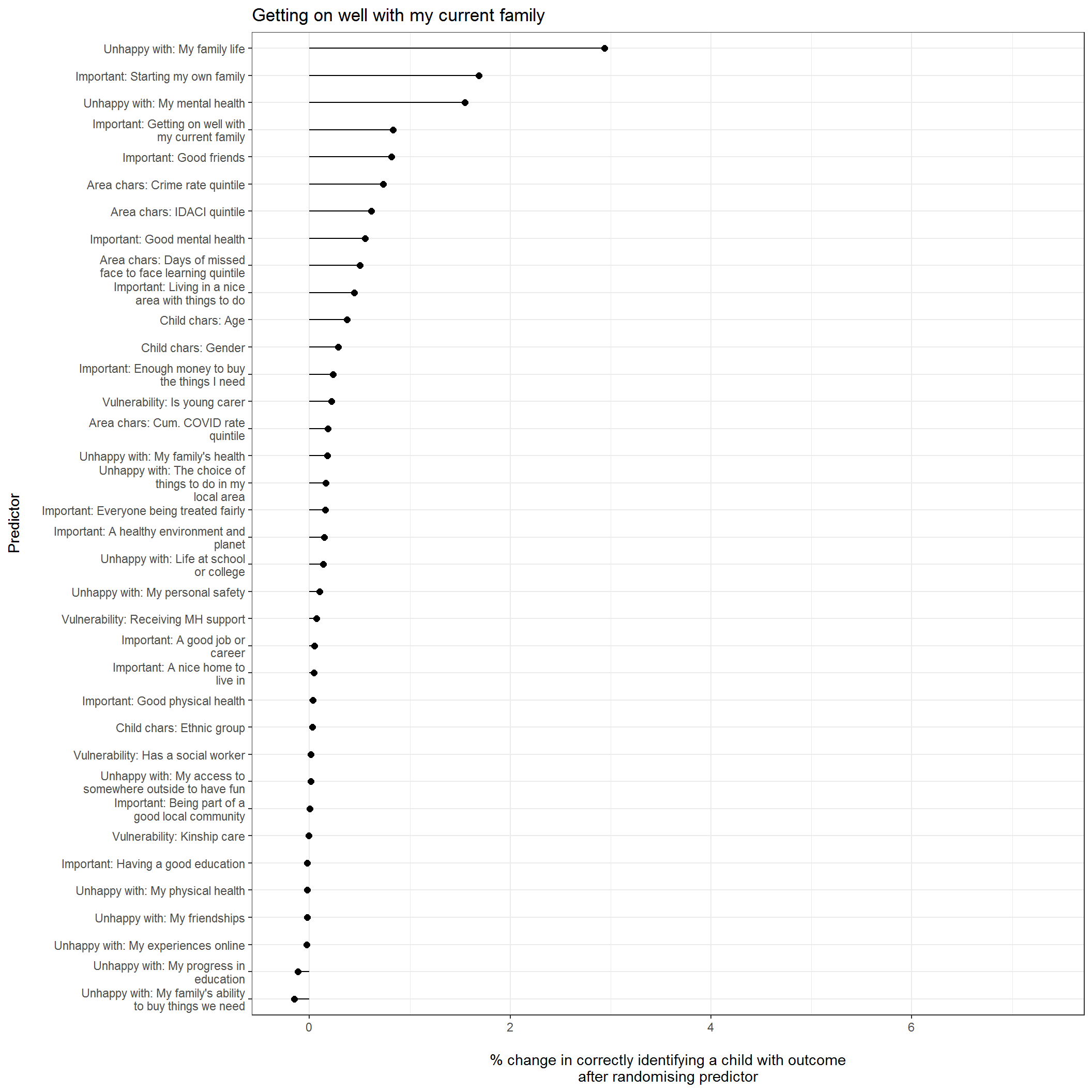Odds ratios

Table below below presents odds ratios for each predictor in the model after controlling for all other predictors included

Overall predictive power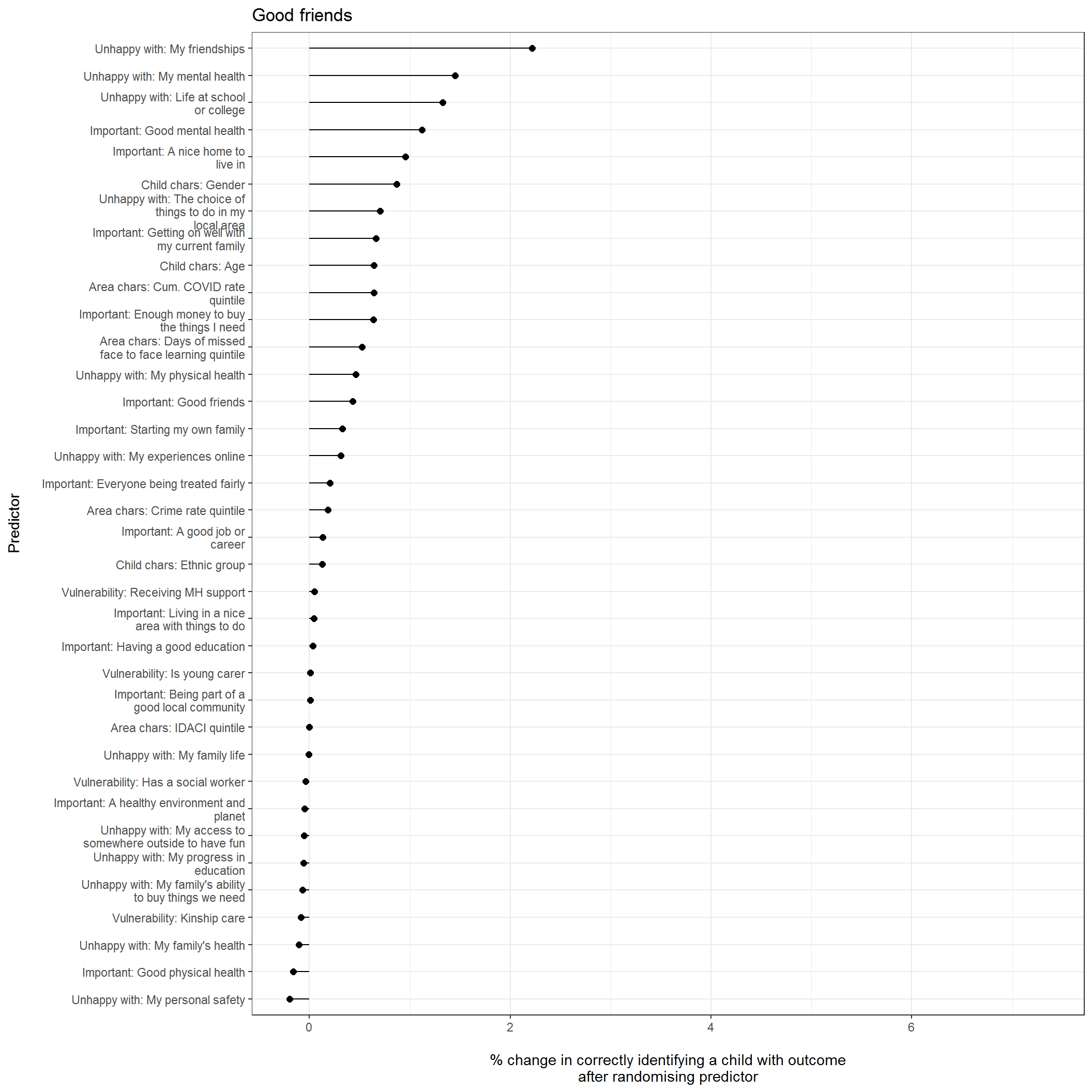Odds ratios

Table below below presents odds ratios for each predictor in the model after controlling for all other predictors included

Overall predictive power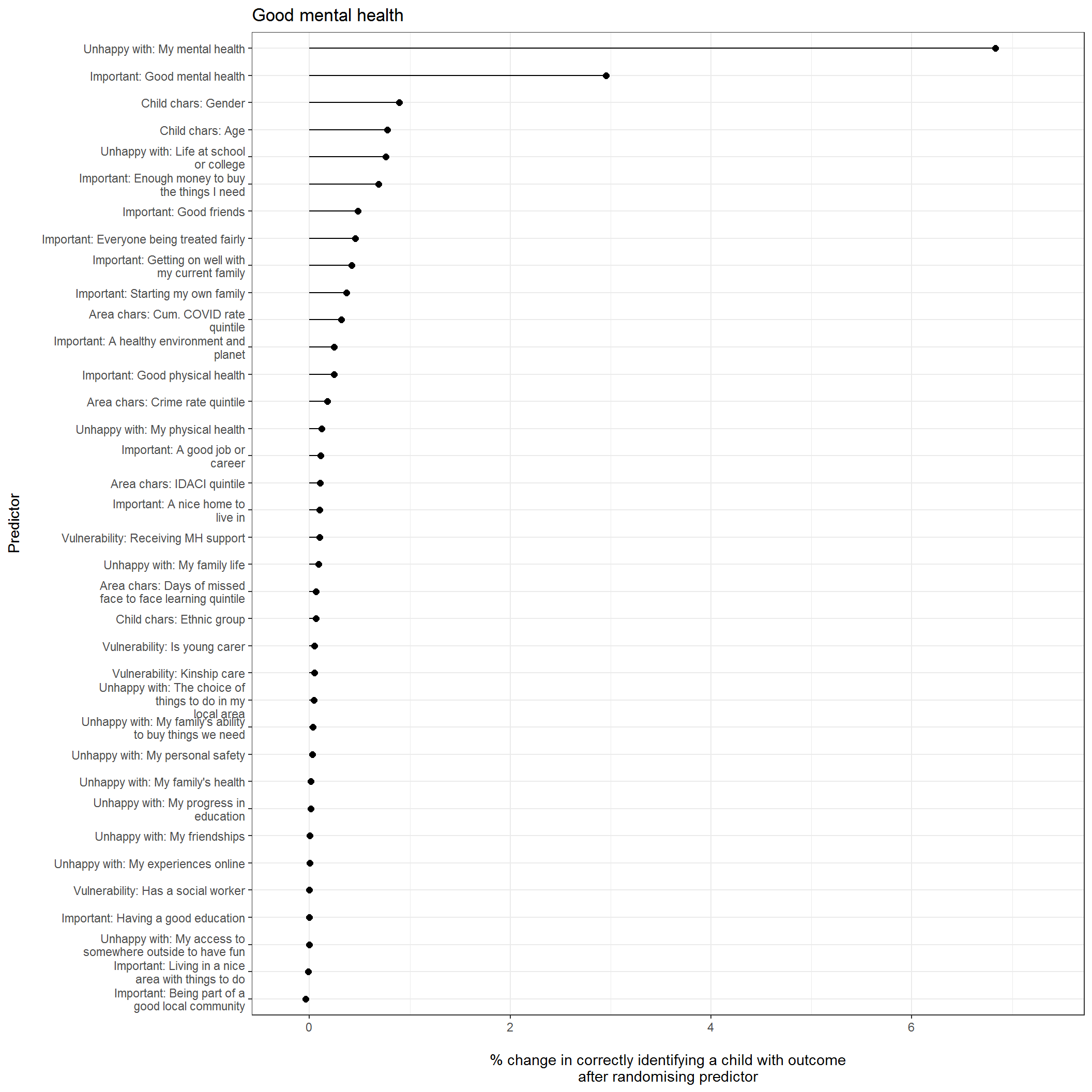Odds ratios

Table below below presents odds ratios for each predictor in the model after controlling for all other predictors included

Overall predictive power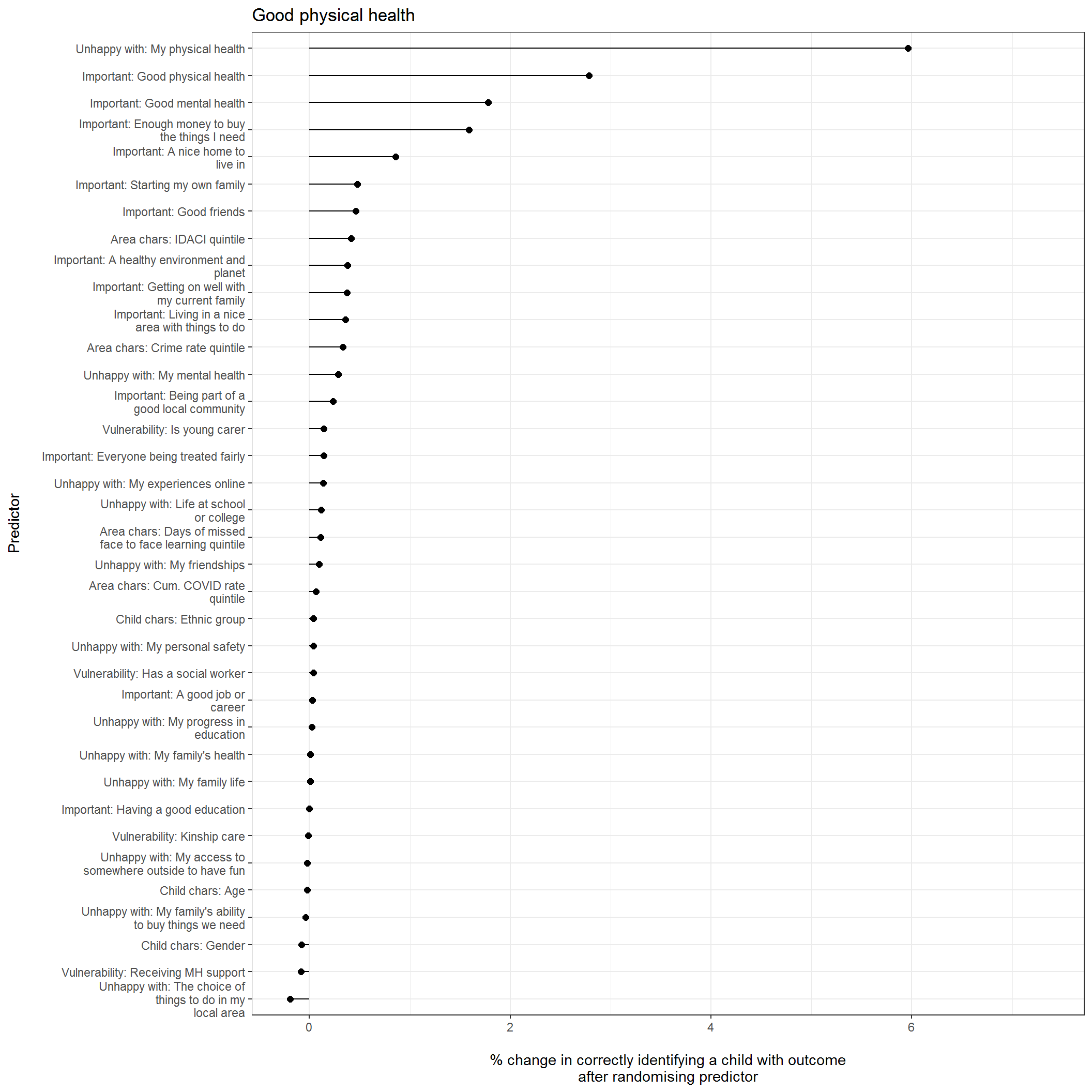Odds ratios

Table below below presents odds ratios for each predictor in the model after controlling for all other predictors included

Overall predictive power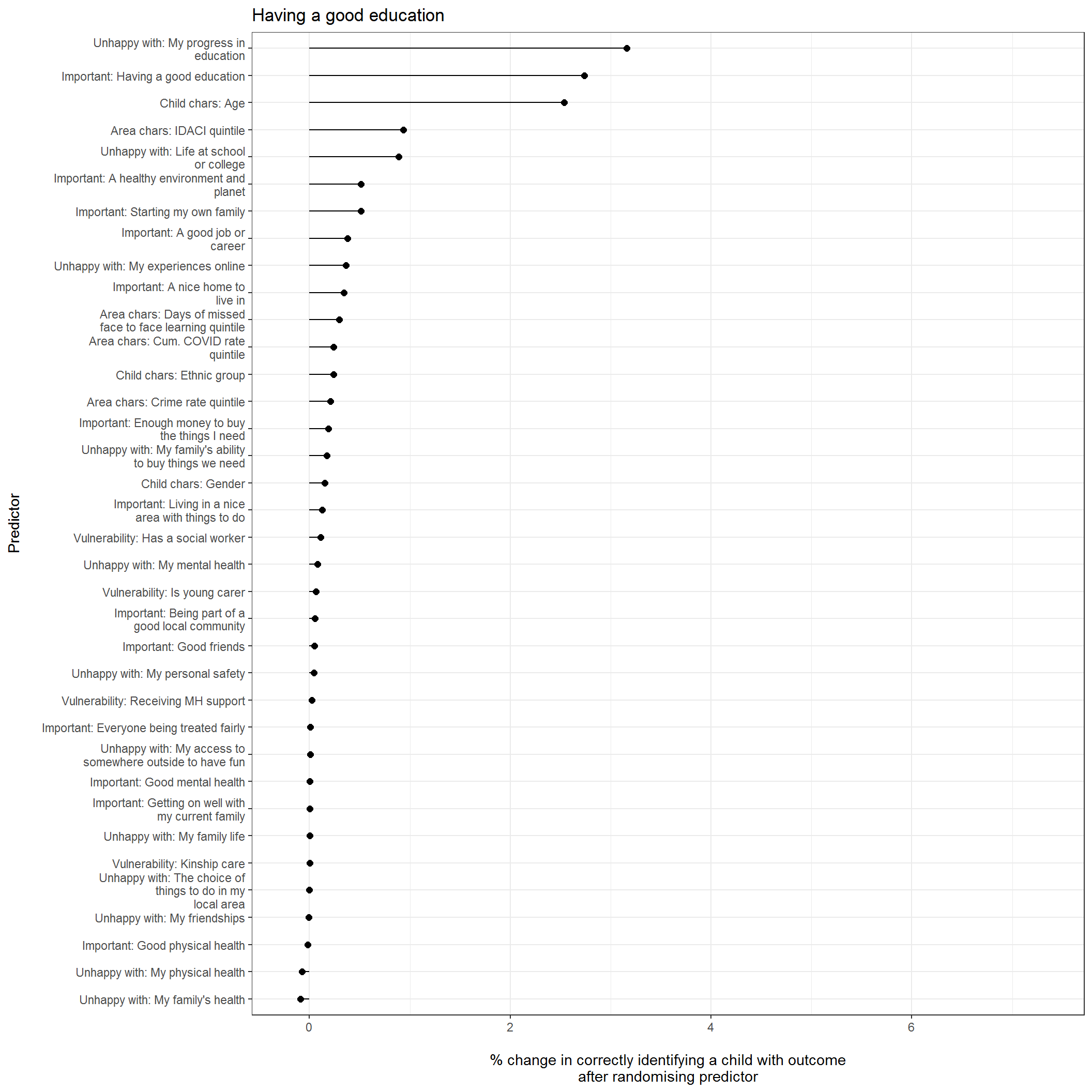Odds ratios

Table below below presents odds ratios for each predictor in the model after controlling for all other predictors included

Overall predictive power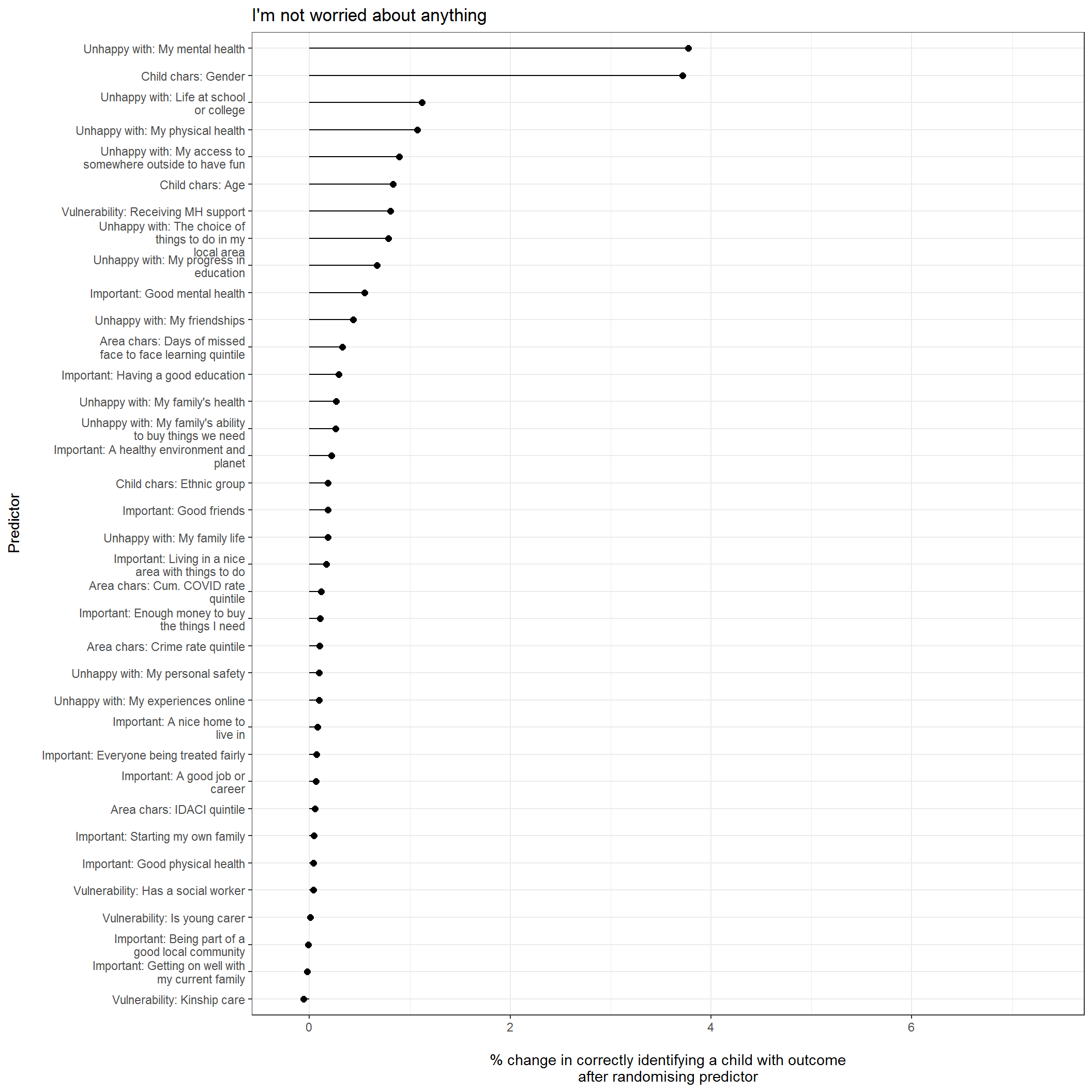Odds ratios

Table below below presents odds ratios for each predictor in the model after controlling for all other predictors included

Overall predictive power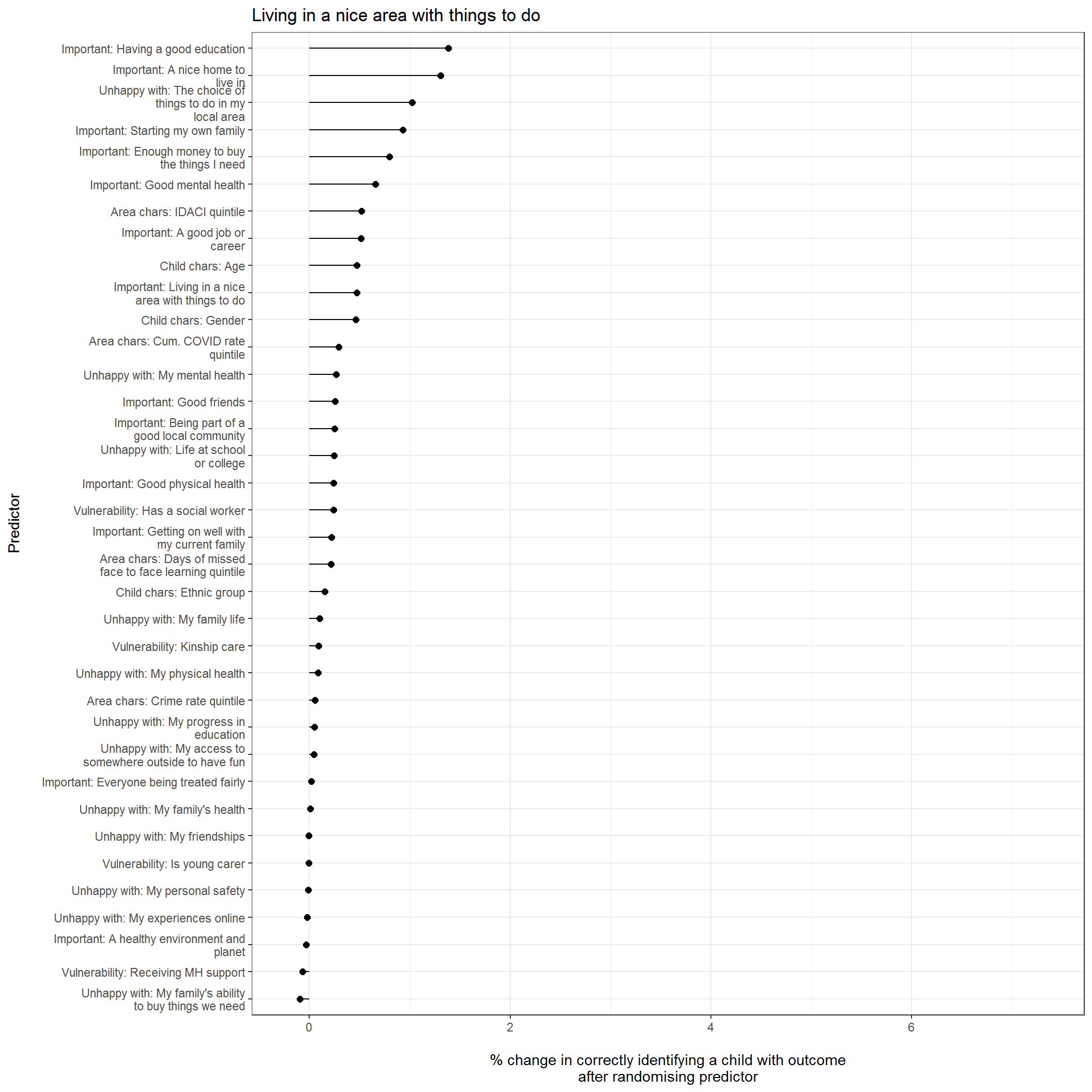Odds ratios

Table below below presents odds ratios for each predictor in the model after controlling for all other predictors included

Overall predictive power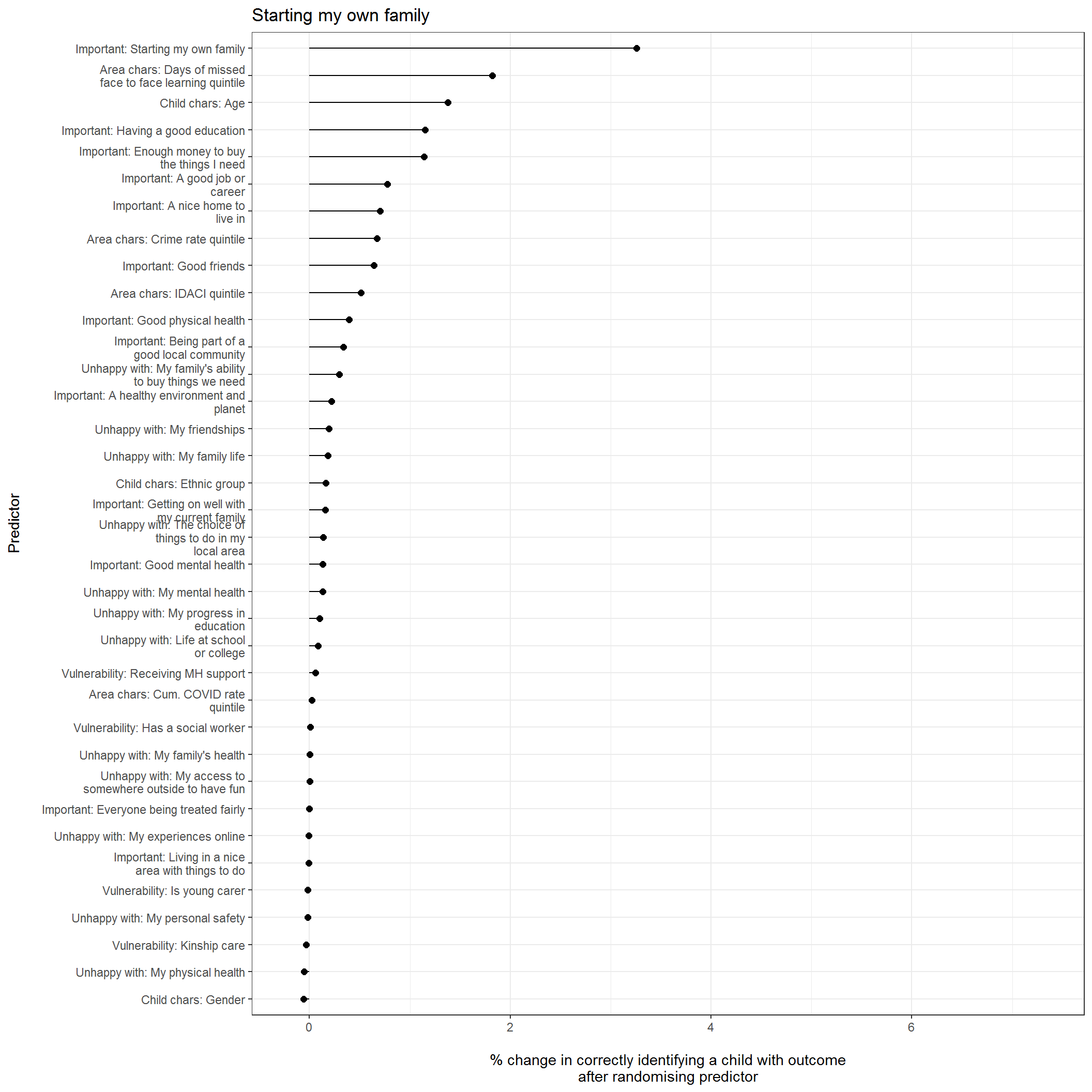Odds ratios

Table below below presents odds ratios for each predictor in the model after controlling for all other predictors included

#### How well can we predict these outcomes overall?